# 4 Digit Subtraction without Regrouping

4 Digit Subtraction without Regrouping – Question 1

Subtract the following: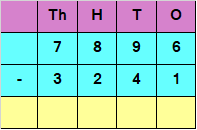Explanation

Subtracting the digits at ones place

6 – 1 = 5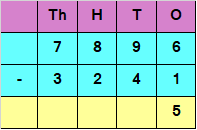Subtracting the digits at tens place

9 – 4 = 5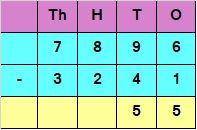Subtracting the digits at hundreds place

8 – 2 = 6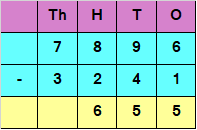Subtracting the digits at thousands place

7 – 3 = 4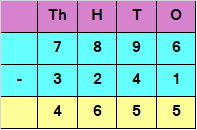Hence, the difference is 4655

4 Digit Subtraction without Regrouping – Question 2

Subtract the following: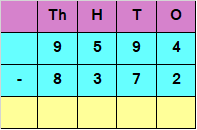Explanation

Subtracting the digits at ones place

4 – 2 = 2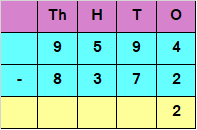Subtracting the digits at tens place

9 – 7 = 2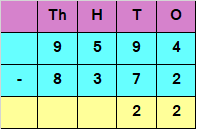Subtracting the digits at hundreds place

5 – 3 = 2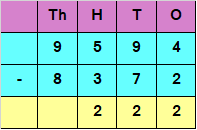Subtracting the digits at thousands place

9 – 8 = 1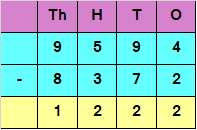Hence, the difference is 1222